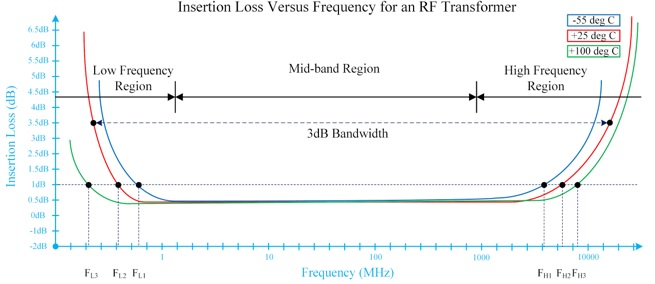Introduction
The first two sections of the Demystifying RF Transformers Series presented a basic overview of the theory and applications for RF transformers and a deeper discussion of RF baluns. This section provides an explanation of the predominant RF transformer performance parameters and data plots representing how these parameters are determined. An understanding of these key parameters is essential when selecting transformers for an application, as well as for comparing different transformer models.

Key RF Transformer Parameters
RF transformers are broadband devices with operating characteristics beyond a narrow set of frequencies. There are several regions where RF transformer performance versus frequency varies from the generally accepted or desired mode. For RF transformers, the desired operating region is called the mid-band region, which is defined by the transformer’s behavior in the low frequency region and high frequency region. In some cases, however, an RF transformer may be used outside the mid-band region depending on design and application requirements. As such, there is sometimes confusion over the exact operating bandwidth for a give RF transformer, as well as the operating conditions under which the specifications of a transformer are derived.

This is why reading the performance plots swept over frequency and temperature is often helpful in determining these factors. One important facet of RF transformer performance presentation is that the insertion loss versus frequency response curves are often presented with a logarithmic scale for frequency and loss (attenuation). It is not uncommon for loss to be represented as a positive quantity, or attenuation presented as a negative value. Therefore, an RF transformer’s loss versus frequency may appear as a bathtub curve or an inverted bathtub curve, depending on presentation (see Figure 1).Figure 11: Average Insertion Loss of a Mini-Circuits TCM1-83X+ RF Transformer

Impedance Ratio
There are several methods of testing RF transformers. Mini-Circuits transformers are tested in balun configurations using a 50Ω impedance system. A Z-conversion (impedance conversion) is either applied to the network analyzer or to the data, using calculations to correct the transformer’s impedance, be it 75Ω, 100Ω, etc. this is done with high performance network analyzers with 50Ω ports.

The impedance ratio is given as X:Y, where X is the secondary port impedance and Y is the primary port impedance. For instance, if the secondary port impedance is 100Ω and the primary port impedance is 50Ω, then the resulting impedance ratio would be 2:1. The primary port is generally designated as the input port, while the secondary port is designated as the output port. Depending on the application or customer requirements, the primary or secondary port designations can be swapped.

Insertion Loss
The insertion loss of an RF transformer is the amount of signal power dissipated or otherwise lost within the device, referenced from the input signal power to the output signal power. All real transformers suffer some insertion loss, and due to the complex nature of RF transformer frequency response, these devices tend to exhibit increased insertion loss at some known upper and lower frequency limits.

The core material for RF transformers has magnetic permeability properties that are a function of temperature. The insertion loss of an RF transformer is sometimes depicted as a plot of insertion loss versus frequency at specific temperatures. The datasheet for a given model usually provides a temperature range where the specific insertion loss is measured.

It is common to see an insertion loss plot of these devices show frequency over a logarithmic scale instead of a linear scale, as transformers are generally broadband devices covering several frequency octaves. For example, Figure 1 illustrates a transformer’s insertion loss performance over frequency at three temperatures.

It is generally desirable for the insertion loss of an RF transformer to be as low as possible within the operating frequency range. The exact insertion loss requirement depends on the circuit. For example, a transformer with high insertion loss in a transmitter signal chain will reduce overall transmitter efficiency. Furthermore, the loss from the signal will dissipate as heat and cause thermally induced performance degradation, or device failure. In a receiver signal chain, an RF transformer with low insertion loss will result in better receiver sensitivity.Figure 12: Average Insertion Loss of a Mini-Circuits TCM1-83X+ RF Transformer
Mid-band Loss
The mid-band region of an RF transformer refers to the range of frequencies at which the device exhibits the lowest insertion loss while staying relatively flat throughout the range. The amount of insertion loss in the mid-band region is commonly referenced as mid-band loss, and is typically the lowest insertion loss for the device. For some broadband applications, mid-band loss is of critical interest, whereas in other applications, an RF transformer may be used at operating frequencies outside of the mid-band region.

It is important to note that the mid-band region is generally not in the “middle” of the observable performance of an RF transformer over frequency, which may be easily misunderstood given the common methods of plotting transformer insertion loss performance over a logarithmic frequency scale.

3 dB Bandwidth
The loss of an RF transformer is often described as being a parabolic frequency response. This means that there is a significant increase in insertion loss at lower and higher ends of the frequency range. It is useful to know when the insertion loss of a transformer is less than a relatively standard amount, such as the commonly used 3 dB point. Hence, the 3 dB bandwidth of an RF transformer is the frequency range at which the insertion loss of the device is less than 3 dB greater than the device’s lowest insertion loss mid-band loss). The 3 dB bandwidth of an RF transformer is variable over temperature, and temperatures outside of the specified operating temperature range may exhibit degradation of the 3 dB bandwidth metrics.